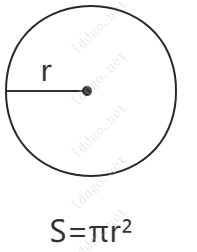•• ARTICLE
• STRING
• CONVERTER
• ENCRYPT
• NETWORK
• MORE
CHART
MATH
• ARTICLE
STRING
CONVERTER
ENCRYPT
NETWORK
MORE
CHART
MATHOnline Tools

# Area Calculator Online

TAG math-tool

the number of digits is reserved after the decimal point, 0 - 10
The number of center angles,unit °
arc length
triangle side length
triangle side length
triangle side length
The number of center angles,unit °
semi-major axis
semi-minor axis
diagonal length
diagonal length
length
width
length
length
height
bottom length
top length
heightRelated Tools
Area Calculator Online-summary

Online area calculator, supports calculating the area of shapes such as circles, fan-shaped, torus, triangles, arches, ellipses, diamonds, rectangles, squares, parallelograms, etc.

Area Calculator Online-instructions

Online area calculator, calculates the area of various shapes and displays the calculation formula for the corresponding shape area.

1. Shape : Choose to calculate the area of what shape. This tool supports calculating the area of shapes such as circles, fan-shaped, rings, triangles, arches, ellipses, diamonds, rectangles, squares, parallelograms, trapezoids, etc., and displays schematic diagrams and formulas for calculating the corresponding shape area.
2. Precision : the number of digits is reserved after the decimal point for the result, optional.
3. After selecting the appropriate shape, enter the corresponding parameters according to the requirements. Click Calculate to get area result. Please be careful to follow the parameter prompts and enter the parameters correctly.
4. There are no specific units for the calculated area, the area units depend on the units of the input parameter.
5. Formula for calculating the area of each shape :
1. Circle : S = πr² , where r is the radius and π is pi.
2. Fan-shaped ( angle ) : S = nπr² / 360 , where n is the angle of the center of the circle, r is the radius, and π is pi.
3. Fan-shaped ( arc length ) : S = (1 / 2)rI , where r is the radius and I is the arc length.
4. Ring : S = πr2² - πr1² , where r2 is the outer radius, r1 is the inner radius, and π is pi.
5. Triangle : S² = p(p - a)(p - b)(p - c) , where a, b, c are the length of the three sides of the triangle, p = (a + b + c) / 2 .
6. Arch :
When n < 180° :
triangle side length a = 2r * sin(n / 2) , where n is the angle of the center of the circle and r is the radius of the circle.
triangle height h = r * cos(n / 2) .
fan area S1 = nπr² / 360 .
triangle area S2 = ah / 2 .
area S = S1 - S2 .
When n = 180° :
area S = (1 / 2)πr² .
When n > 180° :
triangle side length a = 2r * sin((360 - n) / 2), where n is the angle of the center of the circle and r is the radius of the circle.
triangle height h = r * cos((360 - n) / 2) .
fan area S1 = nπr² / 360 .
triangle area S2 = ah / 2 .
area S = S1 + S2 .
7. Ellipse : S = πab , where π is pi, a is the semi-major axis length, and b is the semi-minor axis length.
8. Diamond : S = ab / 2 , where a, b are diagonal lengths of diamonds.
9. Rectangle : S = ab , where a, b are the length and width of the rectangle.
10. Square : S = a² , where a is the side length.
11. Parallelogram : S = ah , where a is length, h is height.
12. Trapezium : S = (a + b)h / 2 , where a is the bottom length, b is the top length, and h is the trapezoidal height.